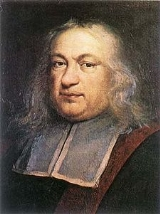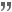xPierre de FermatOverview

Pierre de Fermat was a French
French people
The French are a nation that share a common French culture and speak the French language as a mother tongue. Historically, the French population are descended from peoples of Celtic, Latin and Germanic origin, and are today a mixture of several ethnic groups...

lawyer at the Parlement
Parlement
Parlements were regional legislative bodies in Ancien Régime France.The political institutions of the Parlement in Ancien Régime France developed out of the previous council of the king, the Conseil du roi or curia regis, and consequently had ancient and customary rights of consultation and...

of Toulouse
Toulouse
Toulouse is a city in the Haute-Garonne department in southwestern FranceIt lies on the banks of the River Garonne, 590 km away from Paris and half-way between the Atlantic Ocean and the Mediterranean Sea...

, France
France
The French Republic , The French Republic , The French Republic , (commonly known as France , is a unitary semi-presidential republic in Western Europe with several overseas territories and islands located on other continents and in the Indian, Pacific, and Atlantic oceans. Metropolitan France...

, and an amateur mathematician who is given credit for early developments that led to infinitesimal calculus
Infinitesimal calculus
Infinitesimal calculus is the part of mathematics concerned with finding slope of curves, areas under curves, minima and maxima, and other geometric and analytic problems. It was independently developed by Gottfried Leibniz and Isaac Newton starting in the 1660s...

In the history of infinitesimal calculus, adequality is a technique developed by Pierre de Fermat. Fermat said he borrowed the term from Diophantus. Adequality was a technique first used to find maxima for functions and then adapted to find tangent lines to curves...

. In particular, he is recognized for his discovery of an original method of finding the greatest and the smallest ordinate
Ordinate
In mathematics, ordinate refers to that element of an ordered pair which is plotted on the vertical axis of a two-dimensional Cartesian coordinate system, as opposed to the abscissa...

s of curved lines, which is analogous to that of the then unknown differential calculus
Differential calculus
In mathematics, differential calculus is a subfield of calculus concerned with the study of the rates at which quantities change. It is one of the two traditional divisions of calculus, the other being integral calculus....

, and his research into number theory
Number theory
Number theory is a branch of pure mathematics devoted primarily to the study of the integers. Number theorists study prime numbers as well...

.From what years was Pierre De Fermat a Famous Mathematician?DiscussionsWhere and how was Pierre de Fermat educated?Fermat's primesQuotations

a^p \equiv a \pmod\,\!Also known as Fermat's little theorem|Fermat's little theorem, the formula above is true for any prime p where a

<small> $a^p \equiv a \pmod\,\!$ </small>Also known as Fermat's little theorem|Fermat's little theorem, the formula above is true for any prime $p$ where $a <small> [itex]a^p \equiv a \pmod\,\!$ </small>Also known as Fermat's little theorem|Fermat's little theorem, the formula above is true for any prime $p$ where $a<p$.